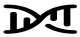# Polygenic risk score (PRS)

Synonyms: Genetic risk score, allele score, risk score, genetic score, genetic values, breeding values

A single variable that is produced by aggregating information from several SNPs that associate with a trait/phenotype and that can be used in prediction or causal analyses (i.e., MR). Usually, genetic risk scores tend to comprise SNPs that have been identified in GWASs at a genome-wide significance p-value threshold to include SNPs that are most robustly associated with a particular trait. PRSs are increasingly being used and tend to include genetic information on the whole genome (or a much less stringent threshold of inclusion). In an unweighted PRS, the number of ‘effect’ alleles each person in a study has are simply added together. For example, if a score was composed from 5 SNPs for a trait and an individual had genotypes of 0, 1, 1, 2 and 0, referring to the number of trait increasing alleles, their score would be 4. Weighted scores multiply the number of ‘effect’ alleles by the magnitude (usually the beta) of the GWAS association value for each SNP. In this example, if the differences in mean level of the trait per allele for each of the 5 SNPs were 0.5, 1.0, 0.5, 2.0 and 0.6, respectively, then the weighted score for the same individual would be 0x0.5 + 1x1.0 + 1x0.5 + 2x2.0 + 0x0.6 = 5.5. Weighted scores are also known as weighted allele sores (WAS).

PRSs have commonly been used in one-sample MR. They can also be generated and used in two-sample MR. In comparison to using each genetic variant as a separate IV, combining them into a single IV can increase statistical power but if some of the variants contributing to the score are invalid (i.e., violate IV assumptions) results are likely to be biased.